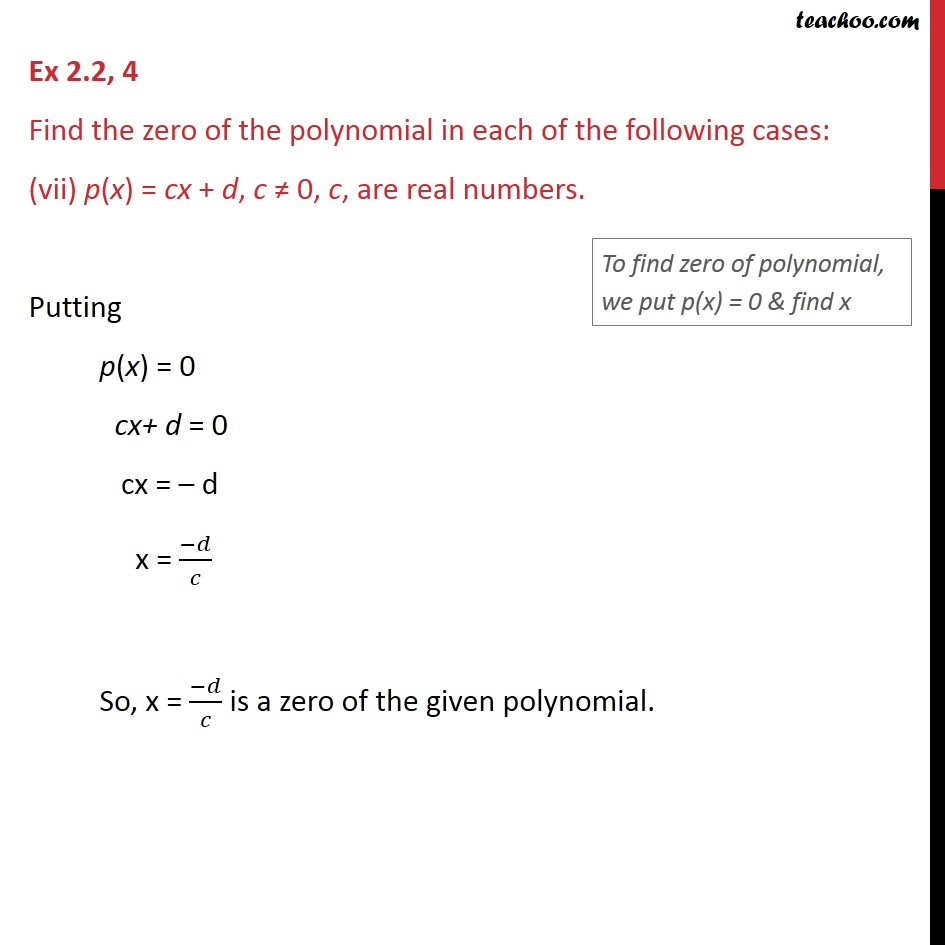Ex 2.2

Chapter 2 Class 9 Polynomials
Serial order wiseLearn in your speed, with individual attention - Teachoo Maths 1-on-1 Class

### Transcript

Ex 2.2, 4 Find the zero of the polynomial in each of the following cases: (vii) p(x) = cx + d, c ≠ 0, c, are real numbers. Putting p(x) = 0 cx+ d = 0 cx = – d x = (−𝑑)/𝑐 So, x = (−𝑑)/𝑐 is a zero of the given polynomial.